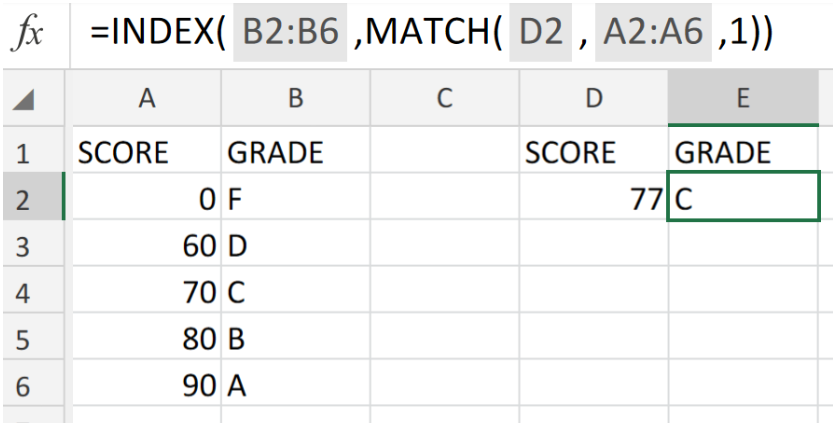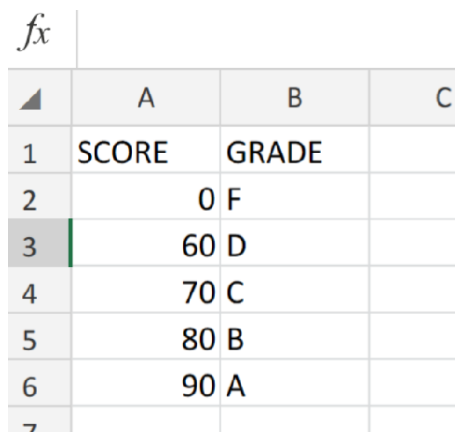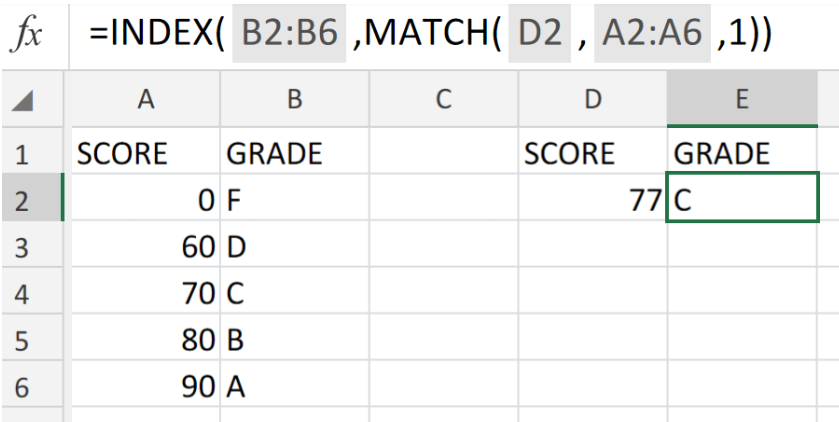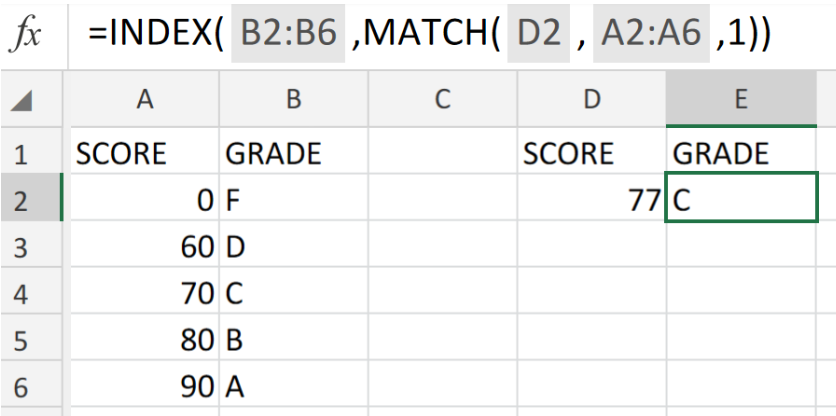Get instant live expert help with Excel or Google Sheets“My Excelchat expert helped me in less than 20 minutes, saving me what would have been 5 hours of work!”

#### Post your problem and you’ll get Expert help in seconds.

Your message must be at least 40 characters
Our professional Expert are available now. Your privacy is guaranteed.

# Basic INDEX MATCH ApproximateFigure 1. of Basic INDEX MATCH Approximate.

In the event that we are required to locate the right row for an approximate matching value in our Excel sheet, we can use the INDEX MATCH functions in Excel. This tutorial will walk through process.

Generic Formula

`=INDEX(grades,MATCH(score,scores,1))`

This operation syntax needs an “approximate match” value, because it is unlikely that the specific score we are searching for can be found in our worksheet table.

## How to use the INDEX MATCH Function in Excel.

We are going to use the INDEX MATCH functions to determine the location of a specific score within a range of scores in our worksheet.

This can be done by following two simple steps!

1. Record and input our range of scores into column in our worksheet. See example illustrated below!Figure 2. of Range of Scores in Excel.

1. Our purpose here is to locate the row for a specific score (77). In the example illustrated below, we are going to enter the following formula syntax into the formula bar for cell E2;

`=INDEX(B2:B6,MATCH(D2,A2:A6,1))`Figure 3. of INDEX MATCH Function in Excel.

The example illustrated above demonstrates how to make use of the INDEX MATCH operation syntax to determine a particular grade from a separate table based on a specific score value.

## Note

• Always be sure to enter the values (column B) where our match is compared to, in ascending order.Figure 4. of Final Result.

## Instant Connection to an Expert through our Excelchat Service:

Our live Excelchat Service is here for you. We have Excel Experts available 24/7 to answer any Excel questions you may have. Guaranteed connection within 30 seconds and a customized solution for you within 20 minutes.

### Did this post not answer your question? Get a solution from connecting with the expert.Another blog reader asked this question today on Excelchat:
Solution examplesindex and match with duplicates. I need to use another column as a reference, so my return value has two match the value of two things for it to return
Solved by E. H. in 60 minsHello, I have a big spreadsheet in which I need to know how many patients came in each month based on provider. I am using the following formula but I still get the N/A error. =INDEX('No Show Appts Data'!D:E, MATCH(1, 'No Show Appts Data'!D:D='No Shows Data'!M2)*('No Shows Data'!E:E='No Shows Data'!N1),0)) No Show Appts Data is the name of the sheet where Column D is Month Year of date in question and Column E is the provider. No Shows Data is the sheet where I am making all the formula calculation where Column M is Month and Year and Column N is the provider in question and therefore M2 is the month in question and N1 the provider in question. How do I fix this error? Thanks
Solved by F. H. in 40 minsI need to find an INDEX function that will convert the Call Day (a number 1-7) to the actual weekday found in row 1 of the DayofWeek named range. I don't know if I'm supposed to use the MATCH function as well or not.
Solved by D. D. in 11 minsI have two columns with names and a third one with email addresses. For every name that matches, I need to copy the email address to an empty column next to the matching name. .
Solved by E. U. in 15 minshelp with INDEX(IndexArea,MATCH(\$M\$5,MatchRows,0),MATCH(\$M\$6,MatchColumns,0))
Solved by Z. Y. in 12 mins## Subscribe to Excelchat.coAnother blog reader asked this question today on Excelchat: